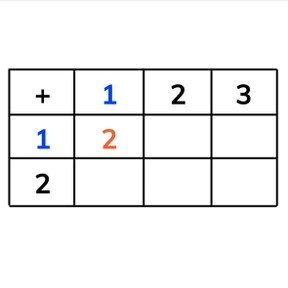Addition to 10 with one more

# Addition to 10 with one more8,000 schools use Gynzy92,000 teachers use Gynzy1,600,000 students use Gynzy

## General

Students learn to add numbers up to 10 by adding one more. They make use of an addition table.

## Relevance

Discuss with students that it is useful if you can add by adding one more. If you know when you can simply add one more, you don't have to calculate different problems separately.

## Introduction

Show the number maze on the interactive whiteboard and ask students if they know which route they should take to go from 1 to 10. They must follow the numbers in sequence. Draw a line from 1 to 2, and continue until you reach 10. Repeat this, but start at 6. Ask students to say what the next number is. Next, show the balls on the interactive whiteboard. Ask students which number comes next and drag the numbers one by one.

## Development

Hold one pencil in each hand. Ask the class how many pencils you are holding in total. Next, hold one pencil in one hand, and two in the other. How many do you now have? Repeat this with different divisions of pencils in which you add 1 more each time. Next, show the caps on the interactive whiteboard. Say that there is one cap already, and that one more is added. Together there are 2. Discuss the problems that follow. Ask the students if they notice anything about the answers. Say that one cap is added each time.

Check that students understand adding numbers up to 10 by adding one more by asking the following questions:
- What do you need to solve first to make completing the addition table easy?
- 2+2 =4. What are the answers to the problems that come next? 2+3 and 2+4?

## Closing

Discuss with students that it is important to be able to add numbers up to 10 by adding one more, so you can quickly solve sets of problems without needing to calculate each one separately. SHow the addition table on the interactive whiteboard and have students discuss in groups which numbers are missing. They must write the numbers down. Ask students how they know which numbers are missing. Ask them if they used any tricks to make determining the numbers easier, or if they calculated each blank space.

## Teaching tips

Students who have difficulty with adding with one more, can make use of MAB blocks or other manipulatives. Have them set out blocks to represent the problem, and then add 1 more and then count the total amount of objects they have.

### The online teaching platform for interactive whiteboards and displays in schools

• Save time building lessons

• Manage the classroom more efficiently

• Increase student engagement